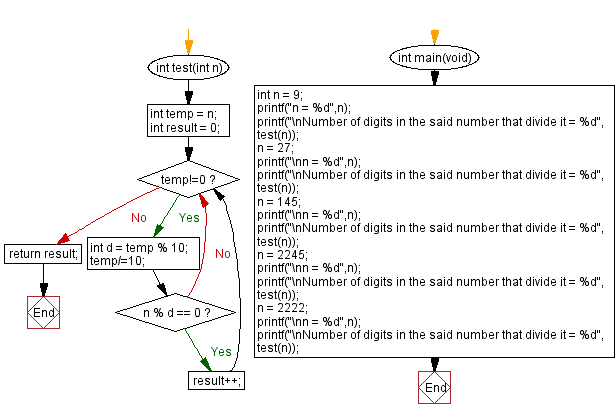﻿ C - Count the digits in a number that divide it

# C Programming: Count the digits in a number that divide it

## C Programming Mathematics: Exercise-33 with Solution

Write a C program to count the digits in a given number that divide it.

Example:
Input (integer value) = 9
Digit of the said number is 9
9 divides 9
So, Output = 1
Input (integer value) = 27
Digits of the said number are 2, 7
2 cannot divides 27
7 cannot divides 27
So, Output = 0
Input (integer value) = 2245
Digits of the said number are 2, 2, 4, 5
2 cannot divides 2245
2 cannot divides 2245
4 cannot divides 2245
5 divides 2245
So, Output = 0

Test Data:
(9) -> 1
(27) -> 0
(145) -> 2
(2245) -> 1
(2222) -> 4

Sample Solution:

C Code:

``````#include <stdio.h>
int test(int n){
int temp = n;
int result = 0;
while(temp!=0){
int d = temp % 10;
temp/=10;
if(n % d == 0)
result++;
}
return result;
}

int main(void) {
int n = 9;
printf("n = %d",n);
printf("\nNumber of digits in the said number that divide it = %d", test(n));
n = 27;
printf("\nn = %d",n);
printf("\nNumber of digits in the said number that divide it = %d", test(n));
n = 145;
printf("\nn = %d",n);
printf("\nNumber of digits in the said number that divide it = %d", test(n));
n = 2245;
printf("\nn = %d",n);
printf("\nNumber of digits in the said number that divide it = %d", test(n));
n = 2222;
printf("\nn = %d",n);
printf("\nNumber of digits in the said number that divide it = %d", test(n));
}
```
```

Sample Output:

```n = 9
Number of digits in the said number that divide it = 1
n = 27
Number of digits in the said number that divide it = 0
n = 145
Number of digits in the said number that divide it = 2
n = 2245
Number of digits in the said number that divide it = 1
n = 2222
Number of digits in the said number that divide it = 4

```

Flowchart:C Programming Code Editor:

Improve this sample solution and post your code through Disqus.

What is the difficulty level of this exercise?

Test your Programming skills with w3resource's quiz.

﻿